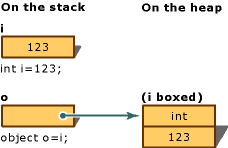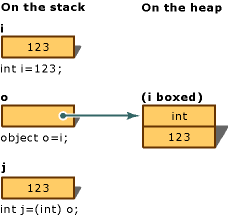# Boxing and Unboxing (C# Programming Guide)

Boxing is the process of converting a value type to the type object or to any interface type implemented by this value type. When the CLR boxes a value type, it wraps the value inside a System.Object and stores it on the managed heap. Unboxing extracts the value type from the object. In the following example, the integer variable i is boxed and assigned to object o.

``````int i = 123;
object o = (object)i;  // boxing
``````

The object o can then be unboxed and assigned to integer variable i:

``````o = 123;
i = (int)o;  // unboxing
``````

## Performance

In relation to simple assignments, boxing and unboxing are computationally expensive processes. When a value type is boxed, a new object must be allocated and constructed. To a lesser degree, the cast required for unboxing is also expensive computationally. For more information, see Performance.

## Boxing

Boxing is used to store value types in the garbage-collected heap. Boxing is an implicit conversion of a value type to the type object or to any interface type implemented by this value type. Boxing a value type allocates an object instance on the heap and copies the value into the new object.

Consider the following declaration of a value-type variable:

``````int i = 123;
``````

The following statement implicitly applies the boxing operation on the variable i:

``````object o = i;  // Implicit boxing
``````

The result of this statement is creating an object reference o, on the stack, that references a value of the type int, on the heap. This value is a copy of the value-type value assigned to the variable i. The difference between the two variables, i and o, is illustrated in the following figure.

Boxing ConversionIt also possible to perform the boxing explicitly as in the following example, but explicit boxing is never required:

``````int i = 123;
object o = (object)i;  // explicit boxing
``````

## Description

This example converts an integer variable i to an object o by using boxing. Then, the value stored in the variable i is changed from 123 to 456. The example shows that the original value type and the boxed object use separate memory locations, and therefore can store different values.

## Example

``````class TestBoxing
{
static void Main()
{
int i = 123;
object o = i;  // Implicit boxing

i = 456;  // Change the contents of i

System.Console.WriteLine("The value-type value = {0}", i);
System.Console.WriteLine("The object-type value = {0}", o);
}
}
/* Output:
The value-type value = 456
The object-type value = 123
*/
``````

The following example demonstrates a case of invalid unboxing and the resulting InvalidCastException. Using try and catch, an error message is displayed when the error occurs.

``````class TestUnboxing
{
static void Main()
{
int i = 123;
object o = i;  // implicit boxing

try
{
int j = (short)o;  // attempt to unbox

System.Console.WriteLine("Unboxing OK.");
}
catch (System.InvalidCastException e)
{
System.Console.WriteLine("{0} Error: Incorrect unboxing.", e.Message);
}
}
}
``````

This program outputs:

Specified cast is not valid. Error: Incorrect unboxing.

If you change the statement:

``````int j = (short) o;
``````

to:

``````int j = (int) o;
``````

the conversion will be performed, and you will get the output:

Unboxing OK.

## Unboxing

Unboxing is an explicit conversion from the type object to a value type or from an interface type to a value type that implements the interface. An unboxing operation consists of:

• Checking the object instance to make sure that it is a boxed value of the given value type.

• Copying the value from the instance into the value-type variable.

The following statements demonstrate both boxing and unboxing operations:

``````int i = 123;      // a value type
object o = i;     // boxing
int j = (int)o;  // unboxing
``````

The following figure demonstrates the result of the previous statements.

Unboxing ConversionFor the unboxing of value types to succeed at run time, the item being unboxed must be a reference to an object that was previously created by boxing an instance of that value type. Attempting to unbox null causes a NullReferenceException. Attempting to unbox a reference to an incompatible value type causes an InvalidCastException.

## C# Language Specification

For more information, see the following sections in the C# Language Specification:

• 4.3.1 Boxing Conversions

## C# Language Specification

For more information, see the following section in the C# Language Specification:

• 4.3 Boxing and Unboxing

#### Concepts

C# Programming Guide

Date

History

Reason

July 2009